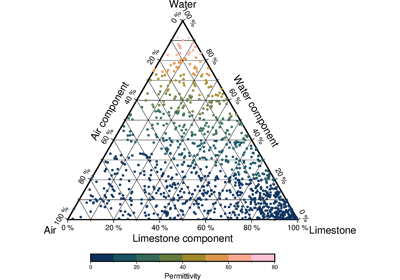# pygmt.Figure.ternary

Figure.ternary(data, alabel=None, blabel=None, clabel=None, *, frame=None, cmap=None, fill=None, width=None, region=None, style=None, timestamp=None, verbose=None, pen=None, panel=None, perspective=None, transparency=None, **kwargs)

Reads (a,b,c[,z]) records from data and plots symbols at those locations on a ternary diagram. If a symbol is selected and no symbol size given, then we will interpret the fourth column of the input data as symbol size. Symbols whose size is <= 0 are skipped. If no symbols are specified then the symbol code (see `style` below) must be present as last column in the input. If `style` is not specified then we instead plot lines or polygons.

Full option list at https://docs.generic-mapping-tools.org/latest/ternary.html

Aliases:

• B = frame

• C = cmap

• G = fill

• JX = width

• R = region

• S = style

• U = timestamp

• V = verbose

• W = pen

• c = panel

• p = perspective

• t = transparency

Parameters
• data (str or list or numpy.ndarray or pandas.DataFrame or xarray.Dataset or geopandas.GeoDataFrame) – Pass in either a file name to an ASCII data table, a Python list, a 2-D `numpy.ndarray`, a `pandas.DataFrame`, an `xarray.Dataset` made up of 1-D `xarray.DataArray` data variables, or a `geopandas.GeoDataFrame` containing the tabular data.

• width (str) – Set the width of the figure by passing a number, followed by a unit (i for inches, c for centimeters). Use a negative width to indicate that positive axes directions be clock-wise [Default lets the a, b, c axes be positive in a counter-clockwise direction].

• region (str or list) – [amin, amax, bmin, bmax, cmin, cmax]. Give the min and max limits for each of the three axes a, b, and c.

• cmap (str) – File name of a CPT file or a series of comma-separated colors (e.g., color1,color2,color3) to build a linear continuous CPT from those colors automatically.

• fill (str) – Select color or pattern for filling of symbols or polygons [Default is no fill].

• alabel (str) – Set the label for the a vertex where the component is 100%. The label is placed at a distance of three times the MAP_LABEL_OFFSET setting from the corner.

• blabel (str) – Set the label for the b vertex where the component is 100%.

• clabel (str) – Set the label for the c vertex where the component is 100%.

• style (str) – symbol[size]. Plot individual symbols in a ternary diagram.

• pen (str) – Set pen attributes for lines or the outline of symbols.

• timestamp (bool or str) – Draw GMT time stamp logo on plot.

• verbose (bool or str) –

Select verbosity level [Default is w], which modulates the messages written to stderr. Choose among 7 levels of verbosity:

• q - Quiet, not even fatal error messages are produced

• e - Error messages only

• w - Warnings [Default]

• t - Timings (report runtimes for time-intensive algorithms)

• i - Informational messages (same as `verbose=True`)

• c - Compatibility warnings

• d - Debugging messages

• panel (bool or int or list) – [row,col|index]. Select a specific subplot panel. Only allowed when in subplot mode. Use `panel=True` to advance to the next panel in the selected order. Instead of row,col you may also give a scalar value index which depends on the order you set via `autolabel` when the subplot was defined. Note: row, col, and index all start at 0.

• perspective (list or str) – [x|y|z]azim[/elev[/zlevel]][+wlon0/lat0[/z0]][+vx0/y0]. Select perspective view and set the azimuth and elevation angle of the viewpoint. Default is [180, 90]. Full documentation is at https://docs.generic-mapping-tools.org/latest/gmt.html#perspective-full.

• transparency (int or float) – Set transparency level, in [0-100] percent range [Default is 0, i.e., opaque]. Only visible when PDF or raster format output is selected. Only the PNG format selection adds a transparency layer in the image (for further processing).

## Examples using `pygmt.Figure.ternary`Ternary diagram

Ternary diagram